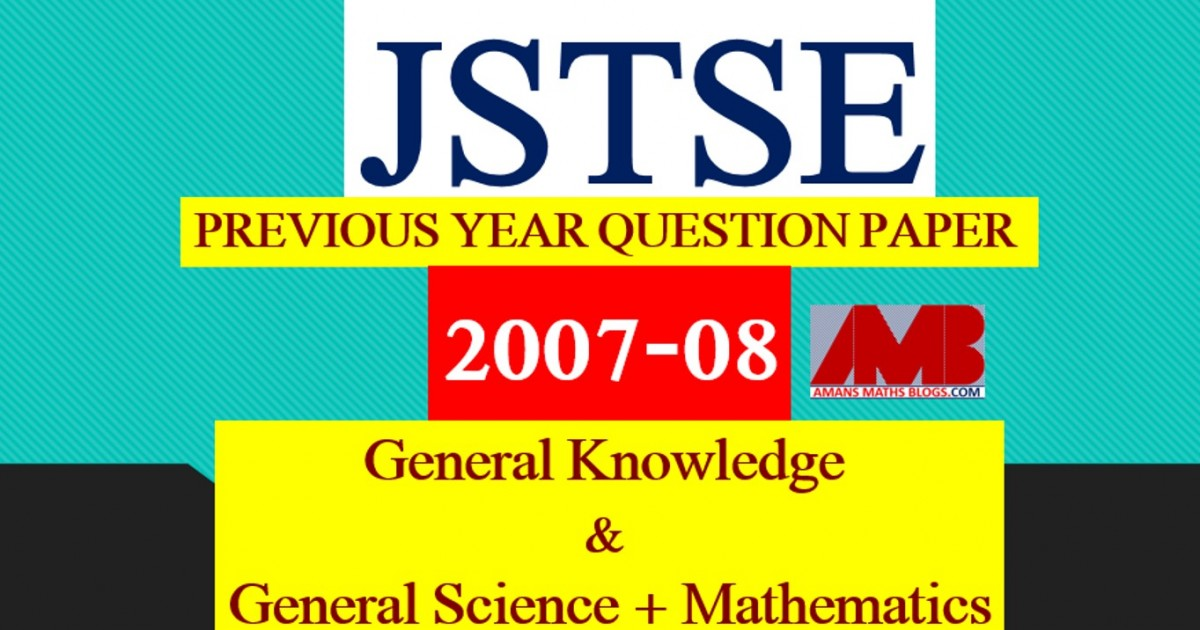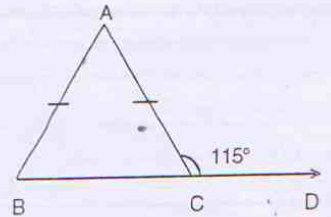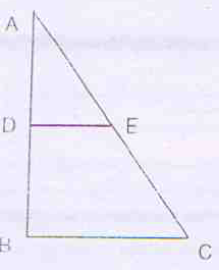Saturday, June 12, 2021
Home > download jstse papers > JSTSE PREVIOUS YEAR QUESTION PAPER 2007-08

# JSTSE PREVIOUS YEAR QUESTION PAPER 2007-08# JSTSE PREVIOUS YEAR QUESTION PAPER 2007-08

In this post, you will get JSTSE Previous Year Question Paper 2007-08.

Junior Science Talent Search Examination (JSTSE)
Junior Science Talent Search Examination (JSTSE) is conducted by Science Branch of DTE. Of Education, Delhi every year usually in the month of January for the students of recognized schools of Delhi.
Eligibility: – Any student of recognized school studying in Class IX in Govt./Govt. Aided/Public/KV/Navodaya/NDMC and must have secured 65% marks in class VIII.

Examination fee: – No examination fee is charged from students for appearing in this exam.
Type of Examination: – The examination consists of two papers.
I. General Knowledge
No. of Questions: 50       Marks : 50     Time Alloted: 50 min
50 50 50 min.
II . General Science and Mathematics
No. of Questions : 150      Marks : 150     Time Alloted: 150 min

No. of Scholarships:- There are 150 scholarships for JSTSE per year.

JSTSE / MVPP Previous Year Questions Papers with Solutions
JSTSE 2020-21 Question Papers with Answer Keys & Solutions
JSTSE 2019-20 Question Papers with Answer Keys & Solutions
JSTSE 2018-19 Question Papers with Answer Keys & Solutions
JSTSE 2017-18 Question Paper Not Available
JSTSE 2016-17 Question Papers with Answer Keys & Solutions
JSTSE 2015-16 Question Papers with Answer Keys & Solutions
JSTSE 2014-15 Question Papers with Answer Keys & Solutions
JSTSE 2013-14 Question Papers with Answer Keys & Solutions
JSTSE 2012-13 Question Papers with Answer Keys & Solutions
JSTSE 2011-12 Question Papers with Answer Keys & Solutions
JSTSE 2010-11 Question Papers with Answer Keys & Solutions
JSTSE 2009-10 Question Papers with Answer Keys & Solutions
JSTSE 2008-09 Question Papers with Answer Keys & Solutions
JSTSE 2007-08 Question Papers with Answer Keys & Solutions
JSTSE 2006-07 Question Papers with Answer Keys & Solutions
JSTSE Physics Questions with Answer Keys & Solutions
JSTSE Chemistry Question with Answer Keys & Solutions
JSTSE Biology Question with Answer Keys & Solutions
JSTSE Maths Question with Answer Keys & Solutions

## General Knowledge

Click Here TO Download This File

## General Science + Mathematics

Click Here To Download This File

JSTSE Previous Year Question Paper 2007-08 Ques No 1:

Factors of (x2/2) – (y2/3) are

Options:

A. (x/Root(2) + y/Root(3))(x/Root(2) + y/Root(3))

B. (x/2 + y/3)(x/2 – y/3)

C. (x/Root(2) – y/Root(3))(x/Root(2) – y/Root(3))

D. (x/Root(2) + y/Root(3))(x/Root(2) – y/Root(3))

JSTSE Previous Year Question Papers 2007-08 Ques No 2:

If Root(x / (x + 3)) = 2-1, then the value of x is

Options:

A. 1/6

B. 6

C. 1

D. 3

JSTSE Previous Year Question Paper 2007-08 Ques No 3:

If x + 1/x = 2, then x – 1/x is

Options:

A. -1

B. 0

C. 1

D. 4

JSTSE Previous Year Question Papers 2007-08 Ques No 4:

If Root(3) = 1.732 and Root(5) = 2.236, then the value of 6/(Root(5) – Root(3)) is

Options:

A. 11.904

B. 6.904

C. 3.504

D. 0.504

JSTSE Previous Year Question Paper 2007-08 Ques No 5:

If x = 1/ (2 – Root(3)), then the value of x2 – 4x + 11 is

Options:

A. 10.268

B. 10

C. 0.732

D. 0.268

JSTSE Previous Year Question Papers 2007-08 Ques No 6:

If x = 1 – Root(2), then the value of (x – 1/x)3 is

Options:

A. 8

B. 8 + Root(2)

C. 8 – Root(2)

D. 3 – Root(2)

JSTSE Previous Year Question Paper 2007-08 Ques No 7:

Simplification (16 x 2n+1 – 4 x 2n) / (16 x 2n+2 – 2 x 2n+1) is

Options:

A. 12n / (14n + 1)

B. 12 x 2n / (14 x 2n+1)

C. 2/7

D. 1/2

JSTSE Previous Year Question Papers 2007-08 Ques No 8:

If x4 + 1/x4 = 47, then the value of x2 + 1/x2 is

Options:

A. 23.5

B. 12.85

C. 7

D. 6.85

JSTSE Previous Year Question Paper 2007-08 Ques No 9:

If f(x) =  x3 – 6x2 + 2x – 4 is divided by g(x) = 3x – 1, then the remainder will be

Options:

A. 107/27

B. 4

C. -107/27

D. -7

JSTSE Previous Year Question Papers 2007-08 Ques No 10:

The factors of a – b – a3 + b3 are

Options:

A. a(1 – a2)(1 – b2)

B. b(1 – b2)(1 + a2)

C. (a – b)(a2 – ab + b2)

D. (a – b)(1 – a – ab – b2)

JSTSE Previous Year Question Paper 2007-08 Ques No 11:

Which of the following points does not lie on a circle whose center is at O(0, 0) and radius is 5Root(2):

Options:

A. (7, -1)

B. (-5, 5)

C. (5, -7)

D. (-1, 7)

JSTSE Previous Year Question Papers 2007-08 Ques No 12:

The graph of a equation is a line parallel to x-axis at a distance 5/2 below it. That equation is

Options:

A. 2x + 5 = 0

B. 2y + 5 = 0

C. y = x – 5/2

D. y = x + 5/2

JSTSE Previous Year Question Paper 2007-08 Ques No 13:

In the figure PQ is perpendicular to PS, PQ || SR, If angle SQR = 28 degree and angle QRT = 65 degree, then the values of x and y areOptions:

A. x = 37 and y = 53

B. x = 25 and y = 65

C. x = 28 and y = 62

D. x = 62 and y = 28

JSTSE Previous Year Question Papers 2007-08 Ques No 14:

In the figure angle ACD = 115 degree and AB = AC then angle BAC will beOptions:

A. 75 degree

B. 65 degree

C. 60 degree

D. 50 degree

JSTSE Previous Year Question Paper 2007-08 Ques No 15:

In the figure, DE = (3/4)BC, DE || BC and distance between DE and BC is 3 units. If BC is 6 units, then area of DBCE isOptions:

A. 9.75 sq. units

B. 13.5 sq. units

C. 15.75 sq. units

D. 18.5 sq. units

JSTSE Previous Year Question Papers 2007-08 Ques No 16:

In the figure, O is the center of circle and angle DAB is 50 degree, then the value of x isOptions:

A. 130 degrees

B. 100 degrees

C. 80 degrees

D. 50 degrees

JSTSE Previous Year Question Paper 2007-08 Ques No 17:

A customer have to pay for a shirt with list price Rs. 900 in a sale “50% + 30%” off

Options:

A. Rs. 280

B. Rs. 315

C. Rs. 350

D. Rs. 450

JSTSE Previous Year Question Papers 2007-08 Ques No 18:

Two numbers are in the ratio 3 : 1 and their sum is 28. In order to get the ratio of 1 : 3, then the second number have to increased by

Options:

A. 28

B. 35

C. 56

D. 84

JSTSE Previous Year Question Papers 2007-08 Ques No 19:

A metallic sheet, rectangular in shape is 56 cm long and 36 broad. From each of its corners, a square of side 8 cm is cut off and an open box is made of remaining sheet. The volume of this box is

Options:

A. 16128 sq. cm

B. 11520 sq. cm

C. 8960 sq. cm

D. 6400 sq. cm

JSTSE Previous Year Question Paper 2007-08 Ques No 20:

The following data has been arranged in ascending order 24, 27, 28, 31, 34, x, 37, 40, 42, 45. If the median of the data is 35, then the value of x is

Options:

A. 37

B. 36

C. 35

D. 34

JSTSE Previous Year Question Papers 2007-08 Ques No 21:

The value of x so that the mode of the data is 7.

3, 5, 6, 7, 5, 4, 7, 5, 6, x, 8, 7,

Options:

A. 5

B. 6

C. 7

D. 8

JSTSE Previous Year Question Paper 2007-08 Ques No 22:

The mean of 13 observation is 14. If the mean of first 7 observation is 12 and the mean of last 7 observation is 16, then the 7th observation is

Options:

A. 12

B. 13

C. 14

D. 16

JSTSE Previous Year Question Papers 2007-08 Ques No 23:

Which of the following statements is not true?

Options:

A. A rhombus is also a parallelogram.

B. All primes are odd.

C. In an equilateral triangle, the median is also a angle bisector.

D. For any real numbers x, x2 > = 0

JSTSE Previous Year Question Paper 2007-08 Ques No 24:

In a cyclic quadrilateral ABCD, if ∠A = 3∠C, then the value of ∠A is

Options:

A. 45 degrees

B. 75 degrees

C. 135 degrees

D. 145 degrees

JSTSE Previous Year Question Papers 2007-08 Ques No 25:

The value of (1-1 + 2-1 – 3-1) / 2 is

Options:

A. 11/12

B. 12/11

C. 1/6

D. 2/5

error: Content is protected !!